## 一、window.devicePixelRatio

``````if(window.devicePixelRatio !== 1){
}``````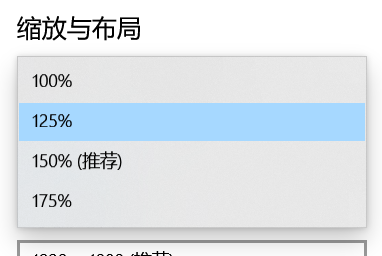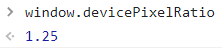## 二、window.outerWidth 与 window.innerWidth

`window.innerWidth`是网页可见区域的宽度，它有一个重要的特点：值会随着页面的缩放变化。页面放大的时候，虽然文字、图片变大了，但我们能看到的内容变少了，也就是可视范围变小了，所以`window.innerWidth`的值会变小，反之同理。

`window.outerWidth`是浏览器窗口的宽度，包含浏览器的边框。窗口在最大化状态下，浏览器左右两边是没有边框的，这个时候，这两个值是相等的：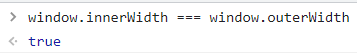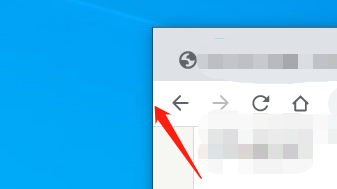``````function isZoom(){
const outerWidth = window.outerWidth,
innerWidth = window.innerWidth;

if(outerWidth - innerWidth > 10) {
}

if(innerWidth - outerWidth > 10) {
}

}``````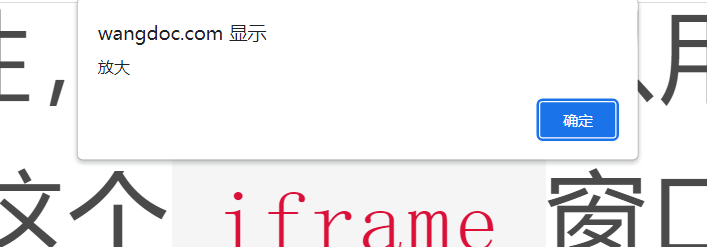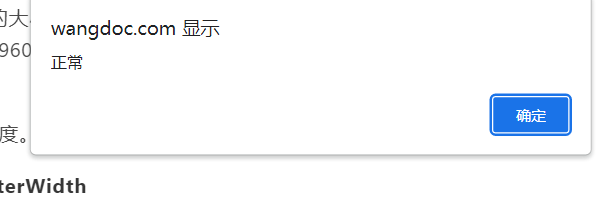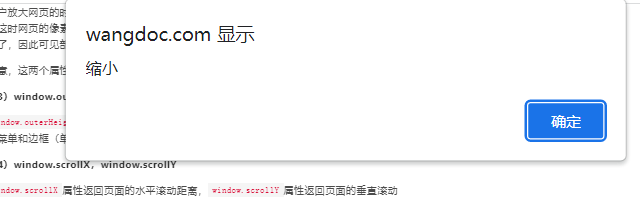End

# 对象和数组

## 利用`Object()`方法来判断一个变量是否为对象：

``````var obj = {};
var isObject = obj === Object(obj);
``````

## 利用`Object.prototype.toString()`方法判断变量类型

`toString`方法返回变量的类型字符串，可以用来判断变量类型。由于数组、字符串、函数、Date等对象(构造函数)都自定义了自己的`toString`方法，所以要使用`Object.prototype.toString()`

``````Object.prototype.toString.call(2); // "[object Number]"
Object.prototype.toString.call(''); // "[object String]"
Object.prototype.toString.call(true); // "[object Boolean]"
Object.prototype.toString.call(undefined); // "[object Undefined]"
Object.prototype.toString.call(null); // "[object Null]"
Object.prototype.toString.call(Math); // "[object Math]"
Object.prototype.toString.call({}); // "[object Object]"
Object.prototype.toString.call([]); // "[object Array]"``````

``typeof []; //'object'``

## 利用`Array.isArray()`判断变量是否为数组

``````typeof []; // 'object'
Array.isArray([]); // true``````

## 关于数组的实例方法`concat()`

“如果数组成员包括对象，concat方法返回当前数组的一个浅拷贝。所谓“浅拷贝”，指的是新数组拷贝的是对象的引用。”
https://wangdoc.com/javascript/stdlib/array.html

``````var arr = [1, 2, {a: 3}];
var arr1 = arr.concat(4); // [1, 2, {a: 3}, 4]
console.log(arr1); // {a: 3}
arr1.a = 5;
console.log(arr);  // {a: 5}``````

## 会改变原数组的方法

`pop` `push` `shift` `unshift` `reverse` `splice`, `sort`

## slice方法

#### 1、不传参数时，会返回原数组的拷贝

``````[1, 2, 3].slice(); // 返回原数组的拷贝 [1, 2, 3]
``````

#### 2、可以将类数组对象转换成整整的数组

``````var arrLikeObj = { 0: 1, 1: 2, length: 2};
Array.prototype.slice.call(arrLikeObj); // [1, 2]``````

## 利用`splice`方法插入新元素

`splice`方法的第二个参数是`0`时，表示只插入新元素，不删除任何元素：

``````var arr = [1, 2, 3];
arr.splice(0, 0, 0);
console.log(arr); // [0, 1, 2, 3]``````

## [JavaScript]使用async函数需要注意的一个问题

async 函数适合执行一些流程，不要试图使用 async 函数返回一些东西。有一个错误的例子就是在Vue的模板中绑定一个计算属性，这个计算属性里面使用 async 函数获取数据。示例代码如下：

HTML模板部分：

``<div>{{computedValue}}</div>``

JavaScript部分：

``````//......
computed: {
async computedValue(){
let val = await aAsyncMethod();
return val;
}
}``````

``'[object Promise]'``

END

## [JavaScript]读取二进制流的内容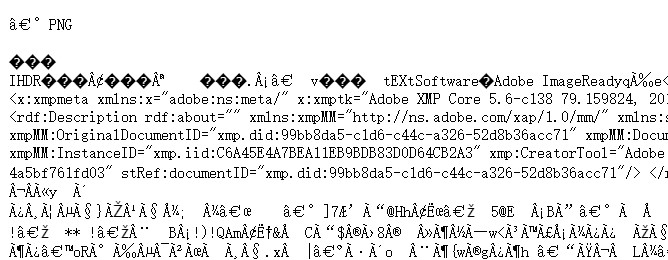axios和请求一个图片为例。

``````request({
//...
responseType: 'arraybuffer'
//...
})``````

``````request({...}).then(r => {
let myblob = new Blob([r], { type: 'image/*' })
})``````

``````request({...}).then(r => {
let myblob = new Blob([r], { type: 'image/*' });
};
})``````

``data:base64; image/*, foiHirf89hHoheruiefhi......``

`fileReader`还提供了`readAsArrayBuffer()``readAsText()`等方法，用来读取不同类型的内容。

# 使用URL.createObjectURL

``````const blob = ......;
const url = URL.createObjectURL(blob);
//生成的url如下：
'blob:http://localhost:9999/0a147e01-97a4-482a-8aa3-e44a2bf79478''``````

``````var link = document.createElement('a');

``URL.revokeObjectURL(url);``

END

## [JavaScript]天天用的for循环竟然不好使了！原因是……

``````const obj1 = [
{ id: 1, ... },
...
];

const obj2 = [
{ xid: 1, value: 1, ...},
...
]``````

``````let i = 0, j = 0, lenI = obj1.length, lenJ = obj2.length;

for (; i < lenI; i++) {
for (; j < lenJ; j++) {
if (obj1[i]['id'] === obj2[j]['xid']) {
obj1[i]['value'] = obj2[j]['value'];
break;
}
}
}``````

``````for (let i = 0, len_i = obj1.length; i < len_i; i++) {
for (let j = 0, len_j = obj2.length; j < len_j; j++) {
if (obj1[i]['id'] === obj2[j]['xid']) {
obj1[i]['value'] = obj2[j]['value'];
break;
}
}
}``````

END

## [HTML]href="###"的作用以及如何设置空链接

``<a href>link</a>``

``<a href="当前页面的URL"></a>``

``<a href="#">link</a>``

``<a href="#idonotcare">link</a>``

``````<a href="##">link</a>

``<a href="javascript:void(0)">link</a>``

`void`总会返回`undefined`，你在`void`之后写啥都没关系。这样写除了要多输入几个字符，就没什么问题了。

``````<a href="javascript:undefined">link</a>

``````<a href="javascript:document.write('0')">link</a>

END

## 1、如何使用

``````let params = {
id: 1
};

if(name){
params.name = name;
}

}``````

``````let params = {
id: 1,
...(name && {name}),
}``````

``````{
id: 1,
name: 'dong',
}``````

``````{
id: 1
}``````

## 2、原理

``name && {name}``

``name && {name: name} //上面是ES6简写形式``

``````if(name){
return {
name: name
}
}else{
return false;
}``````

``...(name && {name})``

``````...(false)
``````

1、如果扩展运算符后面是一个空对象，则没有任何效果
2、如果扩展运算符后面不是对象，则会自动将其转为对象

``...(false)``

``...Object(false)``

``...Boolean {false}``

`Boolean {false}`没有任何属性，所以又等同于

``...{}``

END

## [JavaScript](0, function)()的作用

``````var o = {
f: function(){
console.log(this);
}
}

(0, o.f)();``````

``````o.f();
//{f: ƒ}``````

``````(0, o.f)();
//Window...``````

``````(1, o.f);
(1+1, o.f);
(true, o.f);``````

`()`是不是必须的呢？要调用返回的函数，或者将返回的函数赋值给某个变量，括号就是必须的。下面的代码肯定是不能正确运行的：

``````0, o.f(); //this指向o
var f = 0, o.f //Unexpected token '.'``````

``true && (x = 2)``

END

## [JavaScript]JavaScript获取时间戳的几种方式

JavaScript获取时间戳有多种方式。这里有一个具有纪念意义的时间：

``````var now = new Date('2022/02/02 22:22:22:222');
//Wed Feb 02 2022 22:22:22 GMT+0800 (中国标准时间)``````

#### 方法1：`Date`的`parse`方法

``````Date.parse(now);
//1643811742000``````

`Date.parse`接受一个表示时间的字符串，所以这里的`now`先转换成了字符串`Wed Feb 02 2022 22:22:22 GMT+0800 (中国标准时间)`，然后再被解析成时间戳。因为每个浏览器实现它的方式不一致，所以建议不要用`Date.parse`解析时间字符串。见：Date.parse() - MDN

#### 方法2：`Date实例`的`getTime`方法

``````now.getTime();
//1643811742222``````

#### 方法3：Date实例的valueOf方法

``````now.valueOf();
//1643811742222``````

#### 方法4：`+`操作符获取时间戳

``````+now;
//1643811742222``````

`+`运算符在这里是一元运算符，会将后面的操作数转换成数字，等于`Number()`函数。数字是一种原始值。在JavaScript内部，会调用`toPrimitive`把对象转换成原始值，形式如下：

``toPrimitive(input,preferedType?)``

`preferedType`可选`number``string``+`会将`preferedType`看作是`number`，将按照下面的规则转换：

1. 如果input是原始值，直接返回这个值；
2. 否则，如果input是对象，调用input.valueOf()，如果结果是原始值，返回结果；
3. 否则，调用input.toString()。如果结果是原始值，返回结果；
4. 否则，抛出错误。

`+now`按照规则2调用了`now.valueOf()`

END

- 阅读剩余部分 -